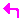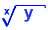Math Functions

Mathematical Functions
logarithms and exponential functions syntax.

This menu is used to format numbers.
You can access the FMT Menu pressing[CST] |FMT|

1) STD
Set number format to standard.
Standard format has 12 digits.
The zeroes after last non-zero digit are not displayed.[CST] |FMT| |STD|

 Example: 3Result: 1.73205080757 Example: 5.0625Result: 2.25

2) FIX
Fix the number format to a pre-definite number, rounding it.[CST] |FMT| |FIX|

Example:

3Result:1.7320508757

Fixing format to 4[CST] |FMT| |FIX|  [ 4 ]

Result:1.7321

3) SCI
Set the number format to scientific mode.[CST] |FMT| |SCI|

Example:

123456789
3[CST] |FMT| |SCI|

Result: 1.23E8

4) ENG
Set the number format to Engeneering mode.
It is similar to SCI, but the exponent is a multiple of 3.[CST] |FMT| |ENG|

 Example 1: 123456789 3[CST] |FMT| |ENG| Result: 123.5E6 Example 2: .0000056 5[CST] |FMT| |ENG| Result:5.600E-6 Example 3: 1200 7[CST] |FMT| |ENG| Result: 1.20000000E3

5) FM
Set the fraction mark to comma "," or dot ".".

6) ML
Show result without break line if clear or
with line breaked if set.

Keyboad Access:[CST] |FMT| |ML|

Example:

(-2,-5)[CST] |FMT| |ML|

 Case 1: 1-If it is set: [ML.] (dot appears) Result: (1.300992853,  -1,92160932647) Case 2: 2-If it is clear: [ML](dot does not appear) Result: (1.300992853,-1,92...) (last numbers are trucated.)

Trigonometric Functions

 1) SIN(X) Returns the sine of an angle Example: 25 (DEG mode set) [SIN] Result: .422618261741 2) COS(X) Returns the cosine of an angle Example: 25 (DEG mode set) [COS] Result: 3.73205080757 3) TAN(X) Returns the tangent of an angle Example: 75 (DEG mode set) [TAN] Result: 3.73205080757 4) ASIN(X) Returns the arc sine of an angle Example: .3 (RAD mode set)[ASIN] Result: .304692654015 5) ACOS(X) Returns the arc cosine of an angle Example: .3 (RAD mode set)[ACOS] Result: 1.26610367278 6) ATAN(X) Returns the arc tangent of an angle. Example: .87 (RAD mode set)[ATAN] Result: .7115991114416

Log Functions
 1) LOG Calculates the decimal logarithim of a number. Example: 8[LOG] Result:.903089986992 2) LN Calculates the natural (Neppier) logarithm of a number. Example: 8[LN] Result:2.07944154168 3) ex Returns e to the X power Example: 5ex Result: 148.413159103 4) 10x Returns the antilog of a number. Example: 510x Result:100000

Inverse, Root and Xroot Functions
1) 1/X
Returns the inverse of a number

Example:

55
1/X

Result:.181818181818E-2

2)Returns the square root of a number.

It is the same that 'XROOT(2,Y)' where Y is a number.

Example:
7Result: 2.64575131106

3)Returns the root X of a number Y
It is the same that 'XROOT(X,Y)',
where X is the root exponent and Y the radicand.

 Example 1: 12 (value for Y) 3 (value for X)Result: 2.28942848511 Example 2: 'XROOT(3,12)' [EVAL] Result: 2.28942848511

4) XY
X at power Y

Example:
5
3
XY

Result:125
( that indeed is equal 53)

 A little step for a man, a step of giant for the mankid. - Neil Armstrong